# How to calculate cumulative angles on specific routes?

1508
0
03-21-2017 08:31 AM
Highlighted

I highly appreciate if someone could provide advice to calculate accurate angles on specific route in a network.

I am trying to calculate accumulative angles from Node 1 to Node 423 on the shortest path as shown in the left side of Figure 1. Network is built from Voronoi poligons which is converted into center line colored green, so it is actually composed of short segments connected each other as shown in the right side of Figure 1 (small nodes indicate edges of each segment).

Figure 1. Network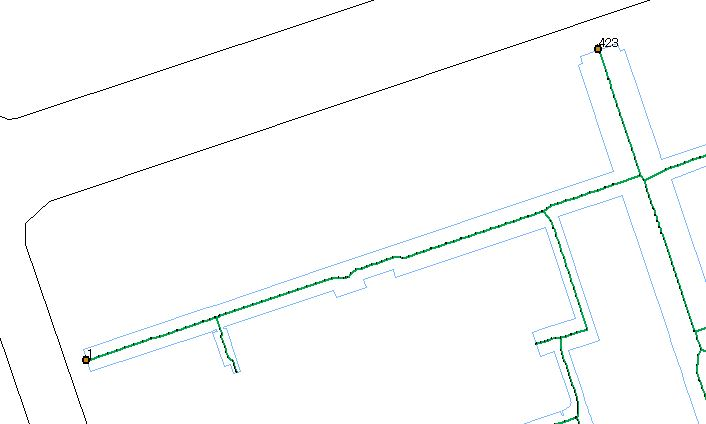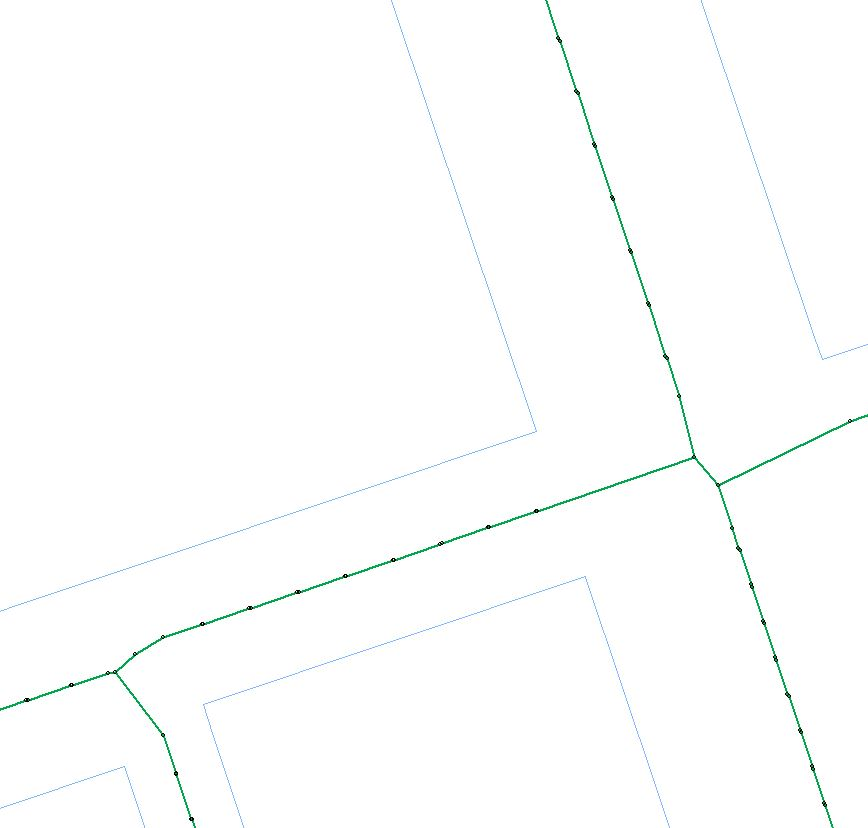Network attributes "Angle" is set as "Unknown" unit (Figure 2).

Source values of "Angle" is set as 0 for all sources (Figure 3).

Only turn penalty is set as "Script" in default values.

Figure 2. Setting of Attributes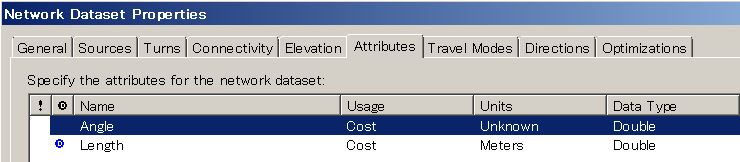Figure 3. Setting of "Source Values"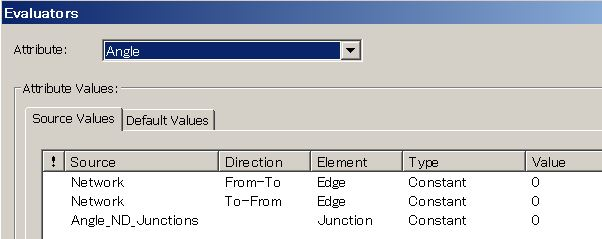Figure 4. Setting of "Default Values"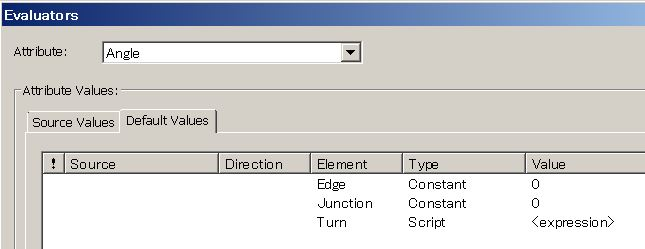VB script is set as below.

====================

Pre-logic Script Code:

turnTime = 0
a = Turn.Angle
If a > 330 And a < 30 Then
turnTime = 0.001 'Straight
ElseIf a > 30 And a < 150 Then
turnTime = 1 'Right-turn
ElseIf a >= 210 And a <= 330 Then
turnTime = 1000 'Left-turn
Else
turnTime=1000000 'U-turn
End If

====================

Value = turnTime

====================

In "OD Cost Matirx", "Length (Meters)" is set as impedance and "Angle" is marked in accumulation tab.

The result accumulative angles (Name = Graphic Pick 1-423) shows 160003000 (Figure 5), which means 160 times of U-turn and 3 times of Left-turn (there are 163 vertices between node 1 to 423).

(Variable "turnTime" should be turnAngle to be precise)

Figure 5. Calculation Result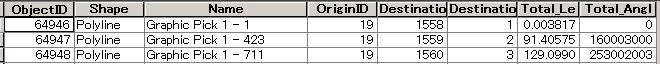However, as shown in the Figure 1, there is no U-turn observed in the network though Left-turn may be possible on the way from 1 to 423 as center line skews at some sections.

Do you have any idea which can fix this problem?

HS

*****Reference*****

For your reference, I also built another simple network to confirm the calculation applying the same setting and obtained correct answer (Figure 6), 1 time of Left-turn and 2 times of Right-turn (total angle = 1002).

Figure 6. Sample Network and Calculation Result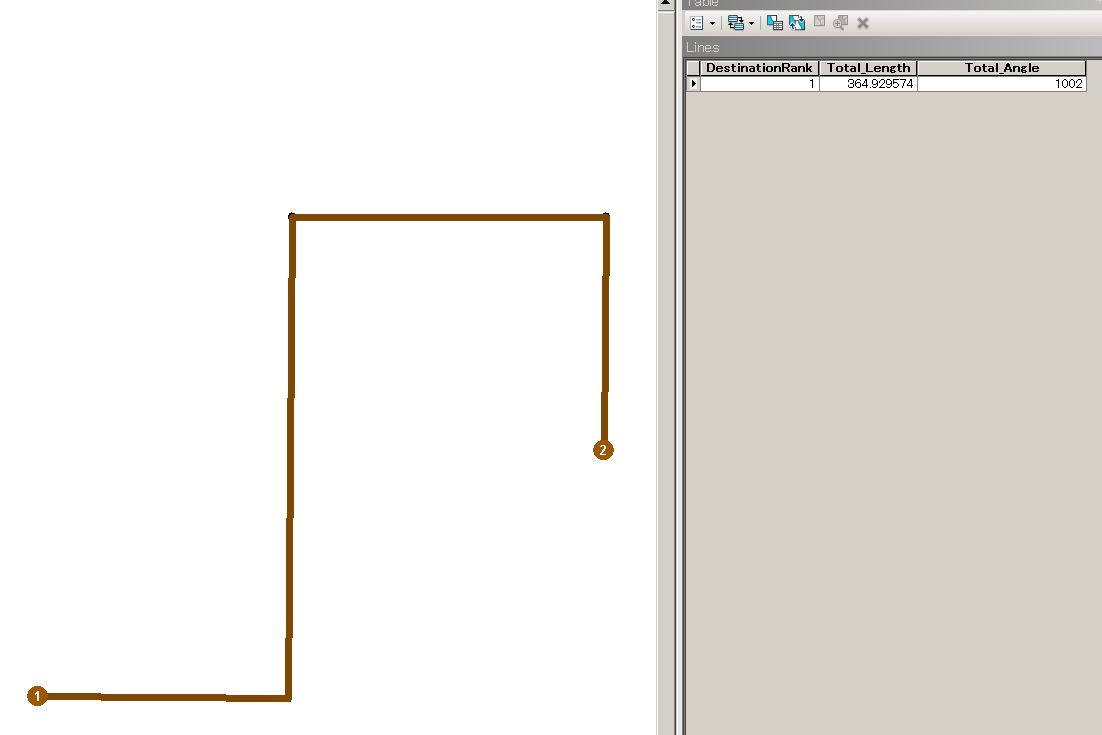The setting itself may be OK, but there may be geometric factors which prevents appropriate calculation in such network composed of very-short segments. Length of the segments distributes from 0.00319 to 3.51153 (Figure 7) which is used as distance impedance (Figure 8), not "Shape_Length" which is automatically calculated when "Network Data Set" is created.

Figure 7. Network Attributes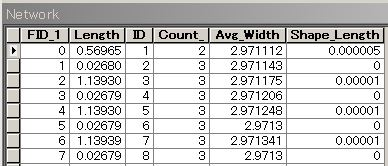Figure 8. Attribute Value of "Length"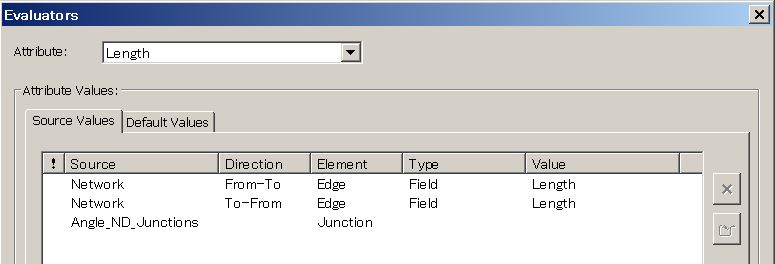Tags (5)
0 Replies